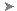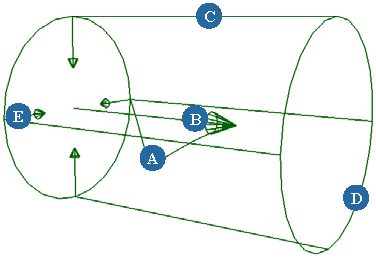# Eddy Property Editor

To create an eddy control object: Choose GetForceEddy from the Simulate toolbar, GetPrimitiveControl ObjectEddy from the other toolbars, or CreateForceEddy from the Hair toolbar.

To redisplay this property editor: Select the eddy object and press Enter.

An eddy force simulates the effect of a vacuum or local turbulence on ICE particles, rigid bodies, hair, and cloth by creating a vortex force field inside a cylinder. The eddy's intensity falls off from the center to the cylinder's border, moving from bottom to top.

Eddy has no effect on Soft body simulations.

For more information on forces in general, see Forces [ Simulation and Effects].

To use this force in an ICE tree, see Creating and Applying ICE Forces to ICE Simulations [ICE Guide].See the parameters in the table below that match the letters in this image.

 Name The name of the force effect. Mute Toggles on/off the force effect. Flow Viscosity The amount of resistance the simulated objects have to the eddy's flow. An eddy force with low viscosity values makes the simulated objects resist motion more strongly, thereby slowing down their flow. Use Size Uses size of the simulated objects in the eddy force's calculations.

## Flow Velocity

The rate of linear motion at which the simulated objects pass through a medium.

 Around Axis (A) The amount of velocity around the cylinder's axis (shown as the number of coils): positive values attract objects toward the cylinder axis. Away from Axis (E) The amount of velocity pushing away from the cylinder's axis. Positive values pushes the objects away from the center, while negative values bring the objects toward it. Along Axis (B) Defines the amount of velocity along the cylinder axis (length of the arrow).

## Influence Zone

 Radius (D) Radius of the eddy's cylinder. Drop Length (C) Length of the eddy's cylinder.

## Decay

 Radial Falloff (decay) along the eddy's Radius starting from its center and moving to its outer edges. A value of 0 is no decay, so a simulated object would have the same amount of influence on it throughout the radius of the cylinder. A value of 1 is a linear decay, so the eddy would have full influence at its center and less influence as the simulated object gets closer to the radius edge where its influence is 0. A value of 2 is the same as 1 except that it produces a quadratic, smooth falloff. Axial Falloff along the eddy's cylinder axis starting from the point of its origin and moving to its end (Drop Length). A value of 0 is no decay, so a simulated object would have the same amount of influence on it throughout the length of the cylinder. A value of 1 is a linear decay, so the eddy would have full influence at its origin and less influence as the simulated object gets closer to the end of the cylinder where its influence is 0. A value of 2 is the same as 1 except that it produces a quadratic, smooth falloff.Except where otherwise noted, this work is licensed under a Creative Commons Attribution-NonCommercial-ShareAlike 3.0 Unported License